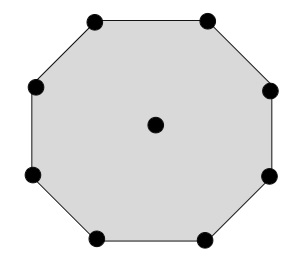# Program for centered nonagonal number in C++

Given with a value ‘n’ and the task is to generate the centered nonagonal number for n and centered nonagonal series till n and display the results.

## What is centered nonagonal number?

Centered nonagonal number contains the nonagonal layers formed by the dots and one corresponding dot in the center.Given above is the figure of centered nonagonal number 𝑁2.It can be calculated using the formula −

$$Nc(n)=\frac{(3n-2)(3n-1)}{2}$$

Input

number: 20

Output

centered nonagonal number : 1711

Input

number: 10

Output

centered nonagonal series : 1 10 28 55 91 136 190 253 325 406

## Algorithm

Start
Step 1→ declare function to calculate centered nonagonal number
int calculate_number(int num)
return (3 * num - 2) * (3 * num - 1) / 2
Step 2→ declare function to calculate centered nonagonal series
int calculate_series(int num)
Loop For int i = 1and i <= num and i++
Print (3 * i - 2) * (3 * i - 1) / 2
End
Step 3→ In main()
Declare int num = 20
Call calculate_number(num)
Declare num = 10
Call calculate_series(num)
Stop

## Example

Live Demo

#include <bits/stdc++.h>
using namespace std;
//calculate centered nonagonal number
int calculate_number(int num){
return (3 * num - 2) * (3 * num - 1) / 2;
}
int calculate_series(int num){
for (int i = 1; i <= num; i++){
cout << (3 * i - 2) * (3 * i - 1) / 2;
cout << " ";
}
}
int main(){
int num = 20;
cout<<"centered nonagonal number : "<<calculate_number(num)<<endl;
num = 10;
cout<<"centered nonagonal series : ";
calculate_series(num);
return 0;
}

## Output

If run the above code it will generate the following output −

centered nonagonal number : 1711
centered nonagonal series : 1 10 28 55 91 136 190 253 325 406